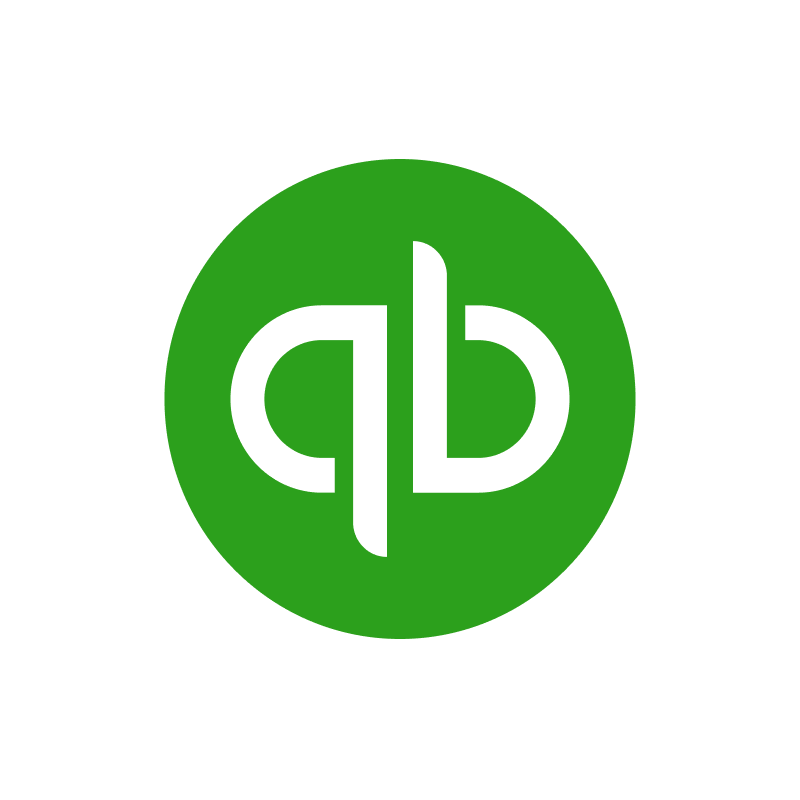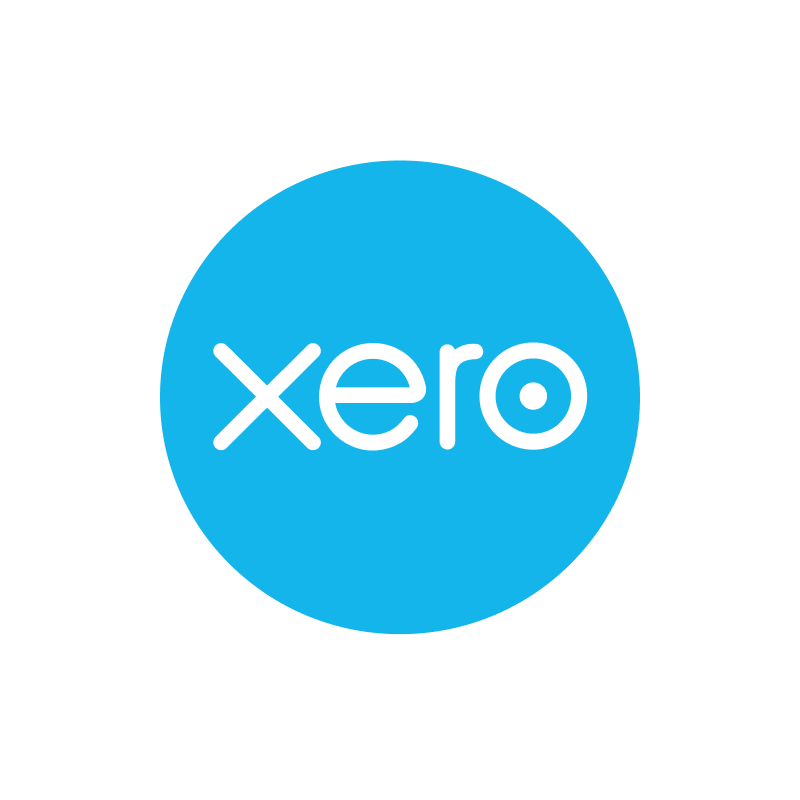The `Address` object is used to represent a contact's or company's address.
Properties

`type`Enum
Optional
The address type. Possible values include: `BILLING`, `SHIPPING`. In cases where there is no clear mapping, the original value passed through will be returned.

`street_1`String
Optional
Line 1 of the address's street.

`street_2`String
Optional
Line 2 of the address's street.

`city`String
Optional

`state`Any
Optional

`country_subdivision`String
Optional

`country`Enum
Optional
The address's country. Possible values include: `AF`, `AX`, `AL`, `DZ`, `AS`, `AD`, `AO`, `AI`, `AQ`, `AG`, `AR`, `AM`, `AW`, `AU`, `AT`, `AZ`, `BS`, `BH`, `BD`, `BB`, `BY`, `BE`, `BZ`, `BJ`, `BM`, `BT`, `BO`, `BQ`, `BA`, `BW`, `BV`, `BR`, `IO`, `BN`, `BG`, `BF`, `BI`, `CV`, `KH`, `CM`, `CA`, `KY`, `CF`, `TD`, `CL`, `CN`, `CX`, `CC`, `CO`, `KM`, `CG`, `CD`, `CK`, `CR`, `CI`, `HR`, `CU`, `CW`, `CY`, `CZ`, `DK`, `DJ`, `DM`, `DO`, `EC`, `EG`, `SV`, `GQ`, `ER`, `EE`, `SZ`, `ET`, `FK`, `FO`, `FJ`, `FI`, `FR`, `GF`, `PF`, `TF`, `GA`, `GM`, `GE`, `DE`, `GH`, `GI`, `GR`, `GL`, `GD`, `GP`, `GU`, `GT`, `GG`, `GN`, `GW`, `GY`, `HT`, `HM`, `VA`, `HN`, `HK`, `HU`, `IS`, `IN`, `ID`, `IR`, `IQ`, `IE`, `IM`, `IL`, `IT`, `JM`, `JP`, `JE`, `JO`, `KZ`, `KE`, `KI`, `KW`, `KG`, `LA`, `LV`, `LB`, `LS`, `LR`, `LY`, `LI`, `LT`, `LU`, `MO`, `MG`, `MW`, `MY`, `MV`, `ML`, `MT`, `MH`, `MQ`, `MR`, `MU`, `YT`, `MX`, `FM`, `MD`, `MC`, `MN`, `ME`, `MS`, `MA`, `MZ`, `MM`, `NA`, `NR`, `NP`, `NL`, `NC`, `NZ`, `NI`, `NE`, `NG`, `NU`, `NF`, `KP`, `MK`, `MP`, `NO`, `OM`, `PK`, `PW`, `PS`, `PA`, `PG`, `PY`, `PE`, `PH`, `PN`, `PL`, `PT`, `PR`, `QA`, `RE`, `RO`, `RU`, `RW`, `BL`, `SH`, `KN`, `LC`, `MF`, `PM`, `VC`, `WS`, `SM`, `ST`, `SA`, `SN`, `RS`, `SC`, `SL`, `SG`, `SX`, `SK`, `SI`, `SB`, `SO`, `ZA`, `GS`, `KR`, `SS`, `ES`, `LK`, `SD`, `SR`, `SJ`, `SE`, `CH`, `SY`, `TW`, `TJ`, `TZ`, `TH`, `TL`, `TG`, `TK`, `TO`, `TT`, `TN`, `TR`, `TM`, `TC`, `TV`, `UG`, `UA`, `AE`, `GB`, `UM`, `US`, `UY`, `UZ`, `VU`, `VE`, `VN`, `VG`, `VI`, `WF`, `EH`, `YE`, `ZM`, `ZW`. In cases where there is no clear mapping, the original value passed through will be returned.

`zip_code`String
Optional

`created_at`DateTime (ISO 8601)
Required

`modified_at`DateTime (ISO 8601)
Required
This is the datetime that this object was last updated by Merge

GET

US
EU

Returns an `Address` object with the given `id`.

Query & Path Parameters

`id`UUID
Required

`include_remote_data`Boolean
Optional
Whether to include the original data Merge fetched from the third-party to produce these models.

`remote_fields`String
Optional
Deprecated. Use show_enum_origins.

`show_enum_origins`String
Optional
Which fields should be returned in non-normalized form.

Field support by integration
See all supported fieldsFreshBooksMoneybirdNetSuiteQuickBooks Online